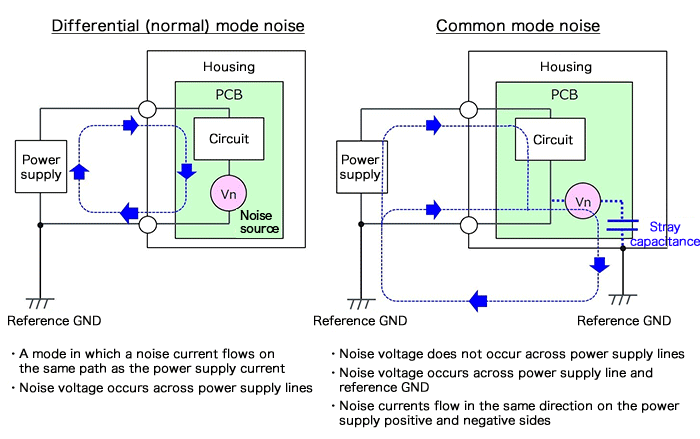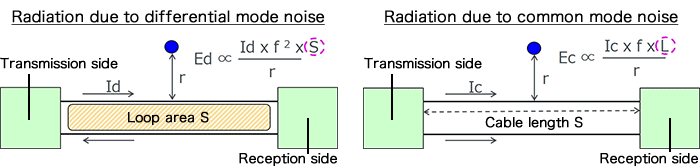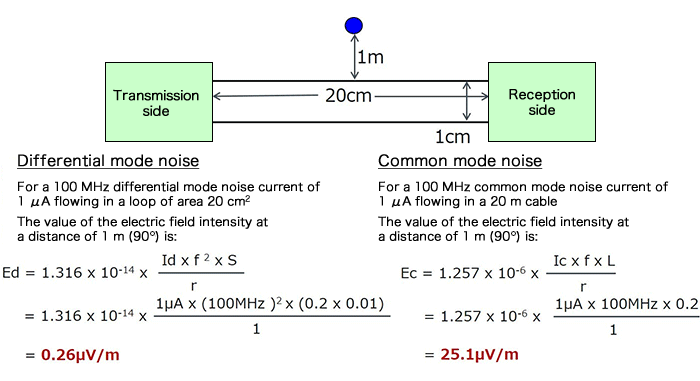# Differential (Normal) Mode Noise and Common Mode Noise－Causes and Measures

2018.03.08

・Electromagnetic interference or EMI is broadly divided into "conducted emission" and "radiated emission ".

・Conducted emission can be further classified into two types, differential (normal) mode noise, and common mode noise.

・With respect to radiation caused by conducted emission, the important factors are the loop area of the line in the case of differential mode noise, and the line length in the case of common mode noise.

・It should be born in mind that under the same conditions, radiation due to common mode noise is far greater than that due to differential mode noise.

In the initial section “What is EMC?“, we explained that electromagnetic interference, EMI, can broadly be divided into two types, “conducted emission” and “radiated emission”. Among these, conducted emission can be further classified into two types, “differential (normal) mode noise” and “common mode noise”, according to the type of conduction. In this section, we explain these latter two types of noise.

## Differential (Normal) Mode Noise and Common Mode Noise

Conducted emission can be classified into two types. One is “differential mode noise”, also known as “normal mode noise”. These names are sometimes used selectively depending on the conditions that apply, but here we shall assume they are the same. The other type is “common mode noise”. These are explained using the following diagrams. Here the discussion is based on power supplies, and so the diagrams are examples in which printed circuit boards (PCBs) with circuits are accommodated within housings, with power fed from outside.In differential mode noise, the noise source appears across power supply lines and is in series with the power supply line, and the noise current flows in the same direction as the power supply current. It is called “differential mode” because the outgoing and return currents are oppositely-directed.

Common mode noise is noise in which a noise current that has leaked via a stray capacitance or the like passes through ground and returns to the power supply line. It is called “common mode” noise because the direction of the noise currents on the positive (+) and the negative (-) sides of the power supply have the same direction. A noise voltage does not appear across the power supply lines.

As explained above, these types of noise are conducted emissions. However, noise currents are flowing in power supply lines, and so noise is radiated.

The electric field intensity Ed of radiation due to differential mode noise can be expressed using the equation below. Id is the noise current in differential mode, r is the distance to the observation point, and f is the noise frequency. Differential mode noise creates a noise current loop, and so the loop area S becomes an important factor. As indicated in the diagram and equation, if the other elements are constant, then for a larger loop area, the electric field intensity is higher.

The electric field intensity Ec of radiation due to common mode noise can be expressed by the equation on the lower right. As the diagram and equation indicate, the cable length L is an important factor.Here, in order to confirm the characteristics of radiation due to the different types of noise, we will insert actual numerical values to calculate*1 the electric field intensities. The conditions in each case are exactly the same. Observed electric field intensities are indicated by blue dots.*1: Equations excerpted from： Detailed Explanation–Electromagnetic Compatibility Engineering, Author: Henry W. Ott, Publisher: John Wiley & SonsOf importance in these calculation results is the fact that, for the same noise current values, the radiation due to common mode noise is far greater (in this example, roughly 100 times greater). In any case, if any of these types of conducted emission and radiated emission that constitute EMI exceed the allowed ranges, noise countermeasures become necessary. In particular, when considering measures to address radiated emission, it should be remembered that measures to deal with common mode noise are particularly important.

Noise countermeasures will hereafter be explained in order, but the most basic measures to deal with noise are, in the case of differential mode noise, decreasing the loop area S, for example by using a twisted-wire cable, and for common mode noise, shortening the cable length insofar as possible. However, constraints on the arrangement and type of components always emerge, and so methods such as adding a filter must also be studied.

Through this section, the reader should have gained an understanding of the different types of noise and their properties.